# Conjugate function

A concept in the theory of functions which is a concrete image of some involutory operator for the corresponding class of functions.

1) The function conjugate to a complex-valued functionis the functionwhose values are the complex conjugates of those of.

2) For the function conjugate to a harmonic function see Conjugate harmonic functions.

3) The function conjugate to a-periodic summable functionon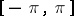is given by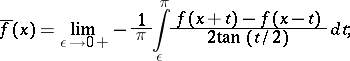it exists almost-everywhere and coincides almost-everywhere with the-sum,, and the Abel–Poisson sum of the conjugate trigonometric series.

4) The function conjugate to a functiondefined on a vector spacedual to a vector space(with respect to a bilinear form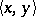) is the function ongiven by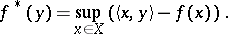(*)

The conjugate of a function defined onis defined in a similar way.

The function conjugate to the function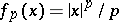,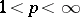, of one variable is given byThe function conjugate to the functionon a Hilbert spacewith scalar productis the function. The function conjugate to the norm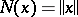on a normed space is the function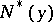which is equal to zero when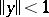and to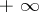when.

Ifis smooth and increases at infinity faster than any linear function, thenis just the Legendre transform of. For one-dimensional strictly-convex functions, a definition equivalent to (*) was given by W.H. Young  in other terms. He defined the conjugate of a function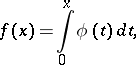whereis continuous and strictly increasing, by the relation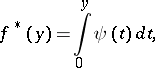whereis the function inverse to. Definition (*) was originally proposed by S. Mandelbrojt for one-dimensional functions, by W. Fenchel  in the finite-dimensional case, and by J. Moreau  and A. Brøndsted  in the infinite-dimensional case. For a convex function and its conjugate, Young's inequality holds: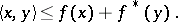The conjugate function is a closed convex function. The conjugation operatorestablishes a one-to-one correspondence between the family of proper closed convex functions onand that of proper closed convex functions on(the Fenchel–Moreau theorem).

For more details see  and .

See also Convex analysis; Support function; Duality in extremal problems, Convex analysis; Dual functions.